# RD Sharma Class 10 Solutions Chapter 2 Polynomials Ex 2.3

## RD Sharma Class 10 Solutions Chapter 2 Polynomials Ex 2.3

These Solutions are part of RD Sharma Class 10 Solutions. Here we have given RD Sharma Class 10 Solutions Chapter 2 Polynomials Ex 2.3

Other Exercises

Question 1.
Apply division algorithm to find the quotient q(x) and remainder r(x) on dividing f(x) by g(x) in each of the following :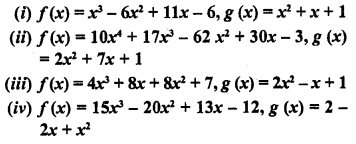Solution: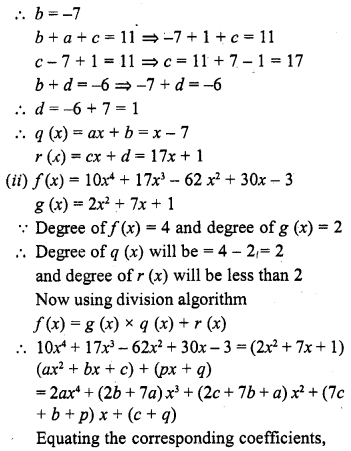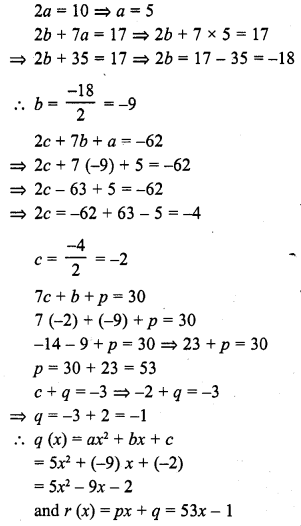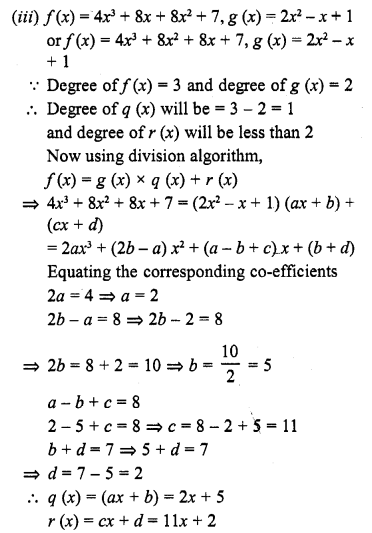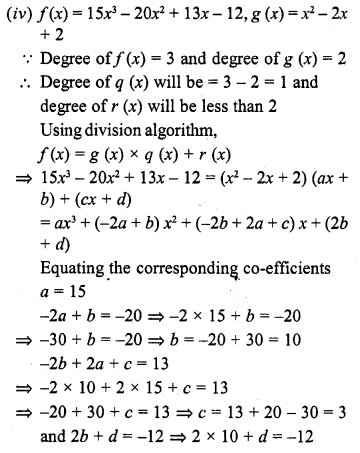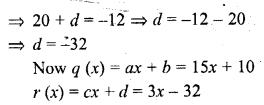Question 2.
Check whether the first polynomial is a factor of the second polynomial by applying the division algorithm.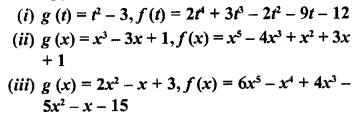Solution: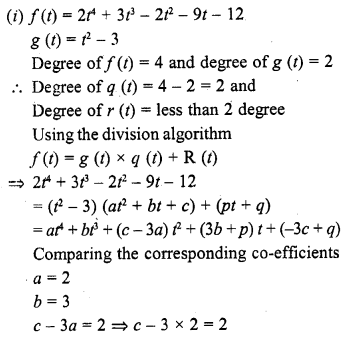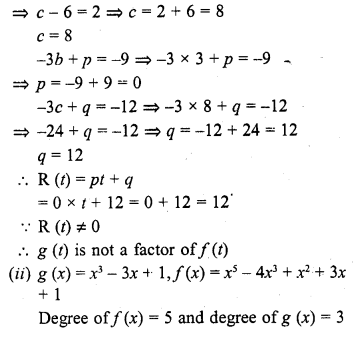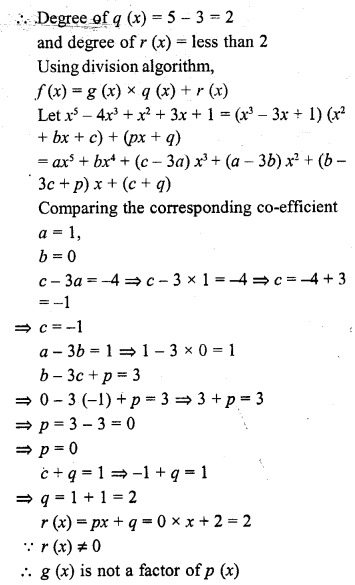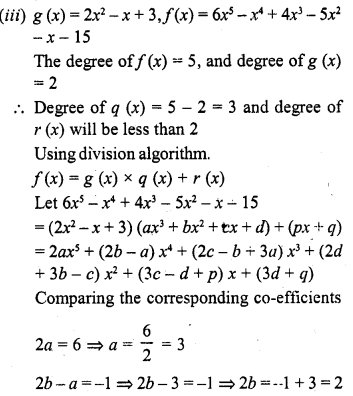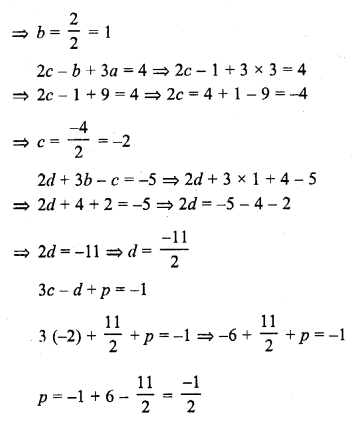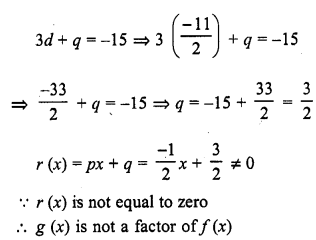Question 3.
Obtain all zeros of the polynomial f(x) = 2x4 + x3 – 14x2 – 19x – 6, if two of its zeros are -2 and -1.
Solution: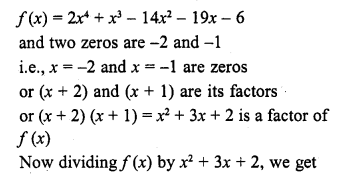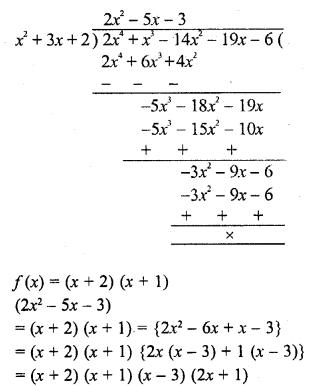Question 4.
Obtain all zeros of f(x) = x3 + 13x2 + 32x + 20, if one of its zeros is -2.
Solution:
f(x) = x3 + 13x2 + 32x + 20
One zero = -2 or x = -2
x + 2 is a factor of f (x)
Now dividing f(x) by x + 2, we get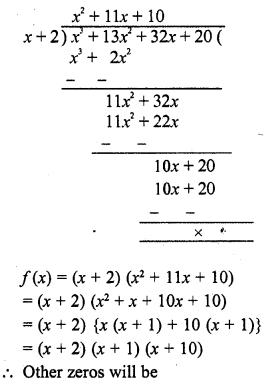x + 1 = 0 => x = -1
and x + 10 = 0
=> x = -10
-1 and -10
Hence zeros are -10, -1, -2

Question 5.
Obtain all zeros of the polynomial f(x) = x4 – 3x3 – x2 + 9x – 6, if two of its zeros are – √3 and √3
Solution: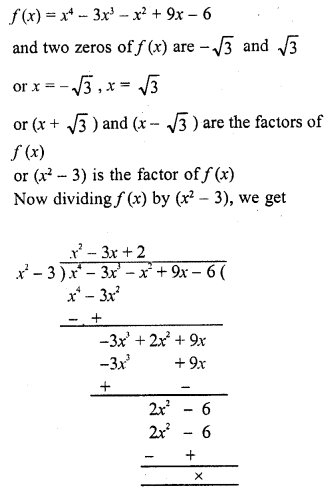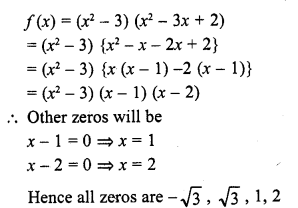Question 6.
Find all zeros of the polynomial f(x) = 2x4 – 2x3 – 7x2 + 3x + 6, if its two zeros are $$\surd \frac { 3 }{ 2 }$$ and – $$\surd \frac { 3 }{ 2 }$$
Solution: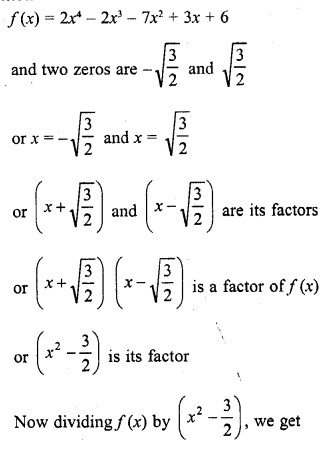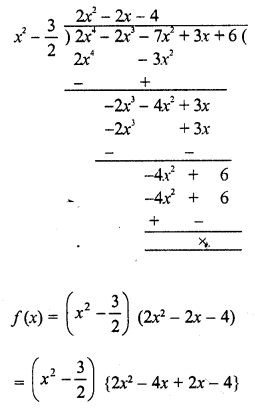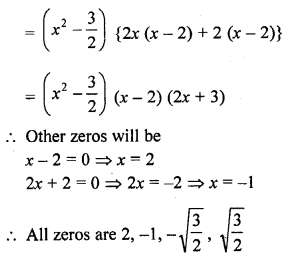Question 7.
Find all the zeros of the polynomial x4 + x3 – 34x2 -4x+ 120, if two of its zeros are 2 and -2. [CBSE 2008]
Solution: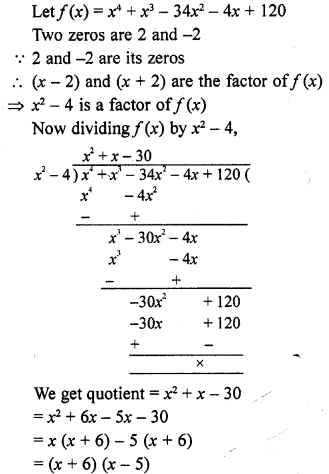Either x + 6 = 0, then x = -6
or x – 5 = 0, then x = 5
Hence other two zeros are -6, 5
and all zeros are 2, -2, -6, 5

Question 8.
Find all zeros of the polynomial 2x4 + 7x3 – 19x2 – 14x + 30, if two of its zeros are √2 and -√2
Solution: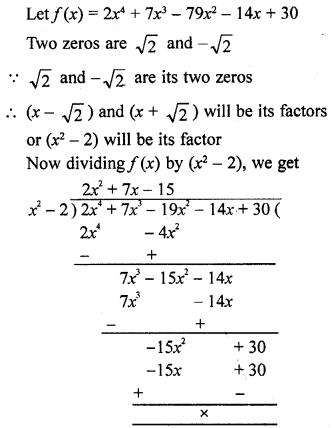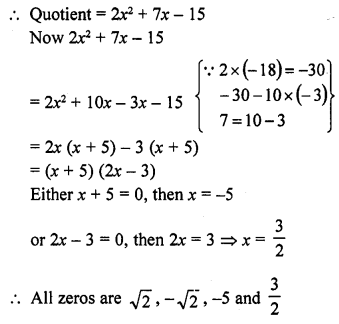Question 9.
Find all the zeros of the polynomial 2x3 + x2 – 6x – 3, if two of its zeros are – √3 and √3. (CBSE 2009)
Solution:
Let f(x) = 2x3 + x2 – 6x – 3
and two zeros of f(x) are – √3 and √3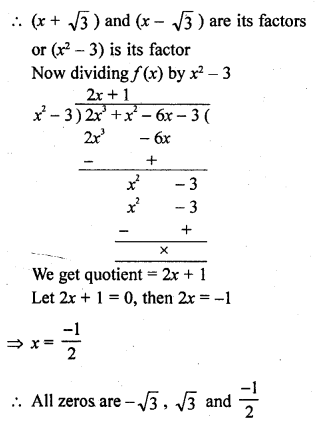Question 10.
Find all the zeros of the polynomial x3 + 3x2 – 2x – 6, if two of its zeros are – √2 and √2 (CBSE2009)
Solution: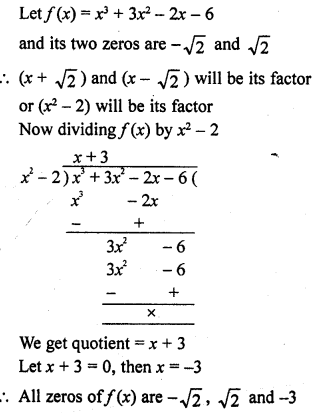Question 11.
What must be added to the polynomial f(x) = x4 + 2x3 – 2x2 + x – 1 so that the resulting polynomial is exactly divisible by x2 + 2x – 3 ?
Solution: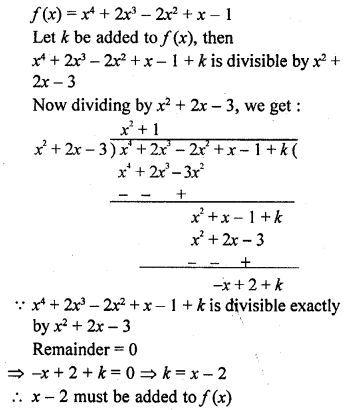Question 12.
What must be subtracted from the polynomial f(x) = x4 + 2x3 – 13x2 – 12x + 21 so that the resulting polynomial is exactly divisible by x3 – 4x + 3 ?
Solution: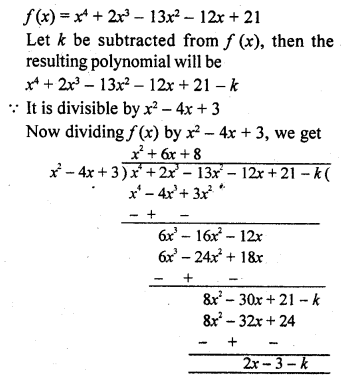The resulting polynomial is exactly divisible by x2 – 4x + 3
Remainder = 0
=> 2x -3 – k = 0
=> k = 2x – 3
(2x – 3) must be subtracted

Question 13.
Given that √2 is a zero of the cubic polynomial 16x3 + √2x2 – 10x – 4√2 , find its other two zeroes. [NCERT Exemplar]
Solution: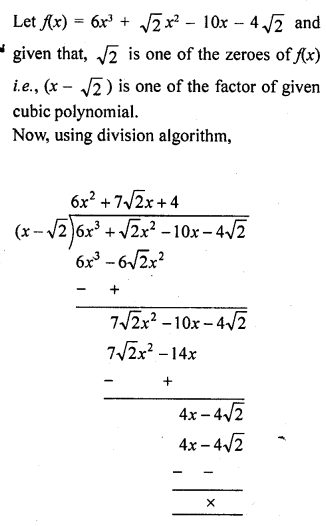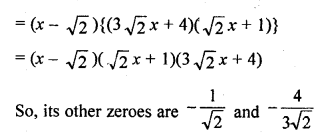Question 14.
Given that x – √5 is a factor of the cubic polynomial x3 – 3 √5 x2 + 13x – 3 √5 , find all the zeroes of the polynomial. [NCERT Exemplar|
Solution: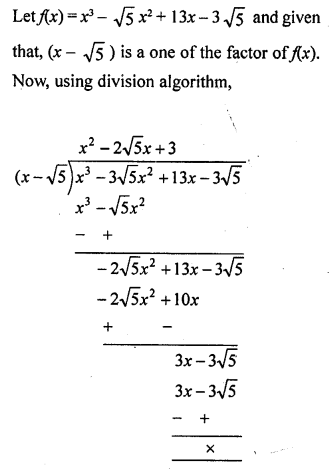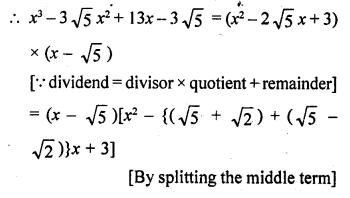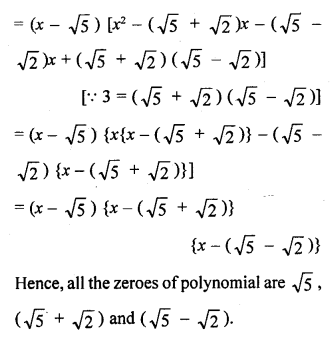Hope given RD Sharma Class 10 Solutions Chapter 2 Polynomials Ex 2.3 are helpful to complete your math homework.

If you have any doubts, please comment below. Learn Insta try to provide online math tutoring for you.